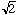Name: Mr William Who is asking: Student Level: Secondary Question: Prove that root 3 is irrational Thanks Hi, I have taken a proof from the notes for a course I teach. The proof is that root 2 is irrational but that should illustrate the procedure. We use what is called a proof by contradiction. The essential idea is that we make an assumption and if we follow that up with a number of logical steps and end up with a false statement, it must be that our assumption was false. So, let's assume thatis rational, in particular assume that= p/q where p and q are relatively prime integers. Thus,= p/q, and hence (squaring both sides) ()2 = (p/q)2 . Equivalently, 2q2 = p2. Here's where we use our number theory sense (on divisibility properties) -- we know 2 | 2q2, thus 2 | p2. But this means that p2 must be even. The only way this can happen is that p itself is even. For simplicity's sake let's write p = 2m. Then we have 2q2 = p2 = 4m2, and q2 = 2m2. But now 2 | 2m2 leading to 2 | q2. The only way this can happen is that q is even also! But then we have both p and q being even when we've already assumed that they were relatively prime. This can't be. Thus, something has gone wrong! What? It can only be our assumption that= p/q where p and q are relatively prime integers.cannot be rational and must be irrational. Cheers, Harley Go to Math Central